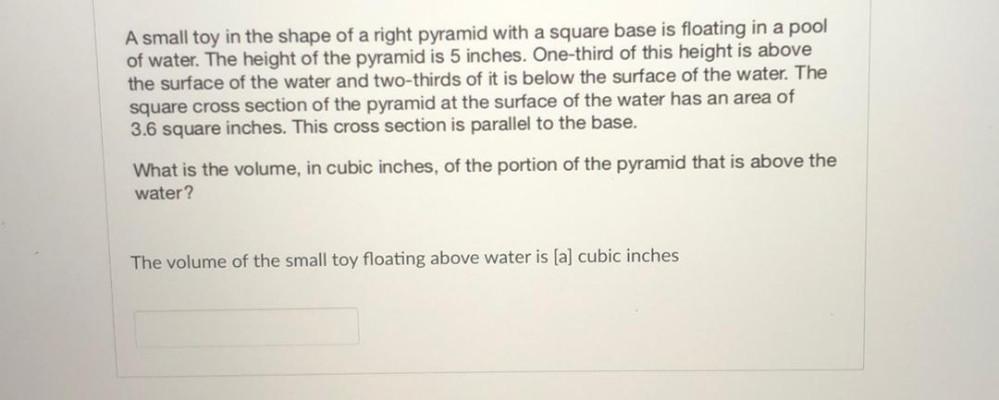Question:

# A small toy in the shape of a right pyramid with a square base is floating in a pool of water. The height of the pyramid is 5 inA small toy in the shape of a right pyramid with a square base is floating in a pool of water. The height of the pyramid is 5 inches. One-third of this height is above the surface of the water and two-thirds of it is below the surface of the water. The square cross section of the pyramid at the surface of the water has an area of 3.6 square inches. This cross section is parallel to the base. What is the volume, in cubic inches, of the portion of the pyramid that is above the water? The volume of the small toy floating above water is (a) cubic inches